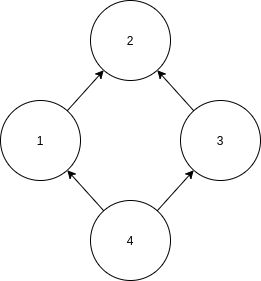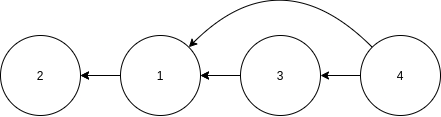Nitrogen Pumpkin Mulch

# npm

## yagl0.3.0 • Public • Published

# YAGLYAGL is Yet Another Graph Library™ for storing and manipulating mathematical graphs. It can be used in a wide range of applications, from package managers that need to find the correct dependencies to network analysis.

Some components haven't thoroughly been tested yet. You are invited to try out this library and report any bugs in the issue tracker.

### Features

• Fully typed graph algorithms including a toplogical sort and a cycle detector
• Algorithms in this library can be paused to only provide part of the result
• Generic graph interface allowing you to define your own graph on which the algorithms will work
• Support for asynchronous iteration protocol, allowing data to be fetched as the algorithm continues.

## Examples

### Sorting a list of dependencies

The following is an example of a simple graph with vertices numbered from 1 to 4.```import { NumberGraph } from "yagl"

const g = new NumberGraph([[1, 2], [3, 2], [4, 1], [4, 3]]);```

If we want to know which node goes before the next, we can use this library to perform a toplogical sort. Note that in the image below all the arrows are now from right to left.This library can lazily calculate the first element that is guaranteed to have no outgoing edges. This can be done like so:

```import { toposort } "yagl"

const ordered = toposort(g);

console.log(ordered.next().value); // outputs 2

// the rest of the items will not be calculated```

If you want to force calculating all elements upfront you can make use of the spread operator:

`console.log([...toposort(g)]) // [2, 1, 3, 4];`

### Using the asynchronus API

The following example demonstrates how to use the asynchronous version of the library to count how many people in a database are connected to one another either directly or indirectly:

```import { AsyncGraph, sccs } from "yagl"

class MyGraphFromDB implements AsyncGraph<Person> {

getOutgoing(person) {
return db.findFriendsOfPerson(person.id);
}

// ...

}

async function printConnectedPeople(person) {
const g = new MyGraphFromDB();
for await (const scc of sccs(g)) {
console.log(`\${scc.length} persons are connected to one another.`);
}
}```

## Graph Types

There are many different graph types possible, each with their own advantages and disadvantages. YAGL comes bundled with a few implementations that are most regularly used. Use the graph type that gives you the best perfomance for your specific application.

Name Edge type Labeled Edge check Add edge Remove edge Incoming Outgoing
StringGraph Directed No O(1) O(1) O(1) O(1) O(1)

## Algorithms

The algorithms are written using ES6 generators, which means that they incrementally perform calculations on-demand. This can be extremely useful if you e.g. only need the first strongly connected component in a graph, or only want to calculate the next task if the previous task has finished.

### preorder(graph)

Performs a depth-first graph traversal starting at the given node. The nodes are traversed in pre-order, meaning that first the node itself is returned, and then its children are visited. Returns an Iterable that generates the next node that has been visited.

### preorderAsync(graph)

Performs a depth-first asynchronous graph traversal starting at the given node. The nodes are traversed in pre-order, meaning that first the node itself is returned, and then its children are visited. Returns an AsyncIterable that generates the next node that has been visited.

### strongconnect(graph)

Finds all strongly connected components in a graph by going through all nodes and edges. Takes `O(|E| + |V|)` time. Returns an Iterable that generates lists of nodes that are strongly connected to one another.

### strongconnectAsync(graph)

Finds all strongly connected components in an asynchronous graph by going through all nodes and edges. Takes `O(|E| + |V|)` time. Returns an AsyncIterable that generates lists of nodes that are strongly connected to one another.

### hasCycle(graph)

Quickly detect whether a given graph has cycles. In the worst case, this method takes `O(|E| + |V|)` time, but it might return faster if there is a cycle.

### hasCycleAsync(graph)

Quickly detect whether a given asynchronous graph has cycles. In the worst case, this method takes `O(|E| + |V|)` time, but it might return faster if there is a cycle.

### toposort(graph)

Performs a topological sort on the graph. Also takes `O(|E| + |V|)` time. Throws an error if the graph contains one or more cycles. Returns an Iterable that generates the next dependency in reverse order.

### toposortAsync(graph)

Performs a topological sort on an asynchronous graph. Also takes `O(|E| + |V|)` time. Throws an error if the graph contains one or more cycles. Returns an AsyncIterable that generates the next dependency in reverse order.

## Keywords

### Install

`npm i yagl`

### Repository

github.com/samvv/YAGL

8

0.3.0